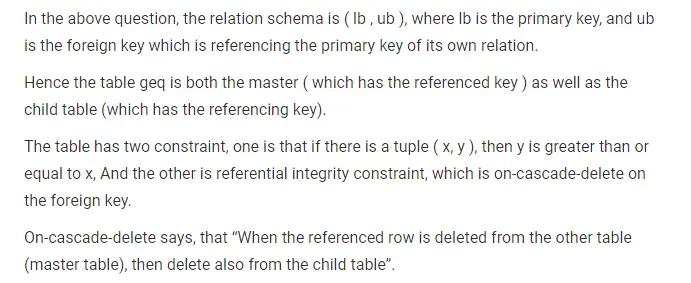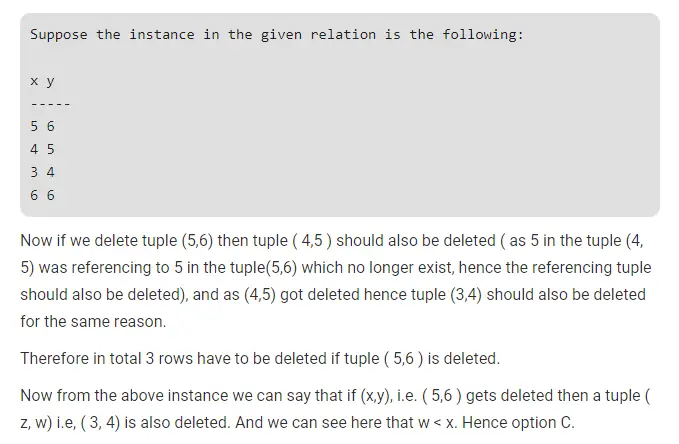# Gate CS-2001 Question Paper With Solutions

Q. 50 Consider a relation geq which represents “greater than or equal to”, that is, (x,y) ∈ geq only if y >= x.

```create table geq
(
ib integer not null
ub integer not null
primary key 1b
foreign key (ub) references geq on delete cascade
)```

Which of the following is possible if a tuple (x,y) is deleted?

(A) A tuple (z,w) with z > y is deleted

(B) A tuple (z,w) with z > x is deleted

(C) A tuple (z,w) with w < x is deleted

(D) The deletion of (x,y) is prohibited

Explanation: